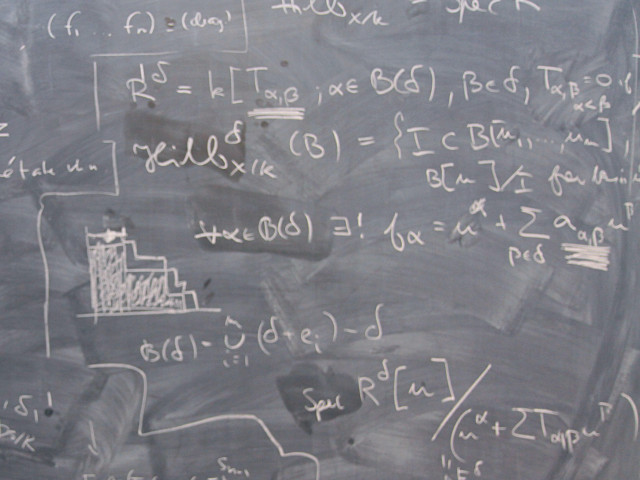Course offering missing for current semester as well as for previous and coming semesters

## Content and learning outcomes

### Course contents

• Mathematics: Complex numbers. Differential equations of first and second order. Separable differential equations. Homogenous and inhomogenous differential equations. Mathematical applications.
• Mechanics: Velocity and acceleration problem. Applications of Newton’s laws.
• Statistics: Descriptive statistics. Probability theory. Conditional probability. Discrete and continuous random variables. The most common density, probability, and distribution functions. Expectation, variance, and standard deviation for descriptive and random variables.

### Intended learning outcomes

The objective of the course is to provide the following abilities:

• Solving linear differential equations, homogeneous and inhomogeneous, of first and second order.
• Solving elementary separable differential equations
• Solving elementary differential equations using integrating factor
• Transferring complex numbers from rectangular form to polar, and vice versa.
• Using algebraic rules applicable to complex numbers
• Representing elementary dynamical problems with differential equations and solving them by hand and by mathematical software
• Simulating elementary mathematical problems using mathematical software
• Applying the classical definition of probability on elementary probability problems
• Selecting applicable probability distribution for well-defined problems
• Applying the central limit theorem
• Solving probability problems using information from complementary events
• Making comparisons between stocastic variables and observed data
• Calculating confidence intervals based on samples acquired from normally distributed populations
• Estimating credibility of calculations

### Course Disposition

No information inserted

## Literature and preparations

### Specific prerequisites

Have a thorough knowledge of mathematics (by means of course HN1901, Mathematics 1) and basic knowledge of the mathematical part of MATLAB (be means of course HM1002, Computerized Tools in the Design Process).

### Recommended prerequisites

No information inserted

### Equipment

No information inserted

### Literature

Matematik för ingenjörer, Staffan Rodhe, Håkan Sollervall

• ISBN: 9789144067964
• Publisher: Studentlitteratur AB
• Country of publication: Sverige
• Edition: 6

Ett stycke statistik, Dag Jonsson, Lennart Norell (paperback)

• ISBN: 9789144029894
• Publisher: Studentlitteratur AB
• Country of publication: Sverige
• Edition: 3

## Examination and completion

If the course is discontinued, students may request to be examined during the following two academic years.

A, B, C, D, E, FX, F

### Examination

• KON1 - Examination, 2,0 hp, betygsskala: A, B, C, D, E, FX, F
• TEN1 - Examination, 3,5 hp, betygsskala: A, B, C, D, E, FX, F
• ÖVN1 - Exercises, 2,0 hp, betygsskala: A, B, C, D, E, FX, F

Based on recommendation from KTH’s coordinator for disabilities, the examiner will decide how to adapt an examination for students with documented disability.

The examiner may apply another examination format when re-examining individual students.

### Other requirements for final grade

Passed written exam in statistics (TEN1), grades A-F.
Passed written exam in complex numbers and differential equations (KON1), grades A-F.
Passed Exercises in mathematic software (MATLAB) and assignments in statistics (ÖVN1), grades A-F.

### Opportunity to complete the requirements via supplementary examination

No information inserted

### Opportunity to raise an approved grade via renewed examination

No information inserted

### Examiner

No information inserted

### Ethical approach

• All members of a group are responsible for the group's work.
• In any assessment, every student shall honestly disclose any help received and sources used.
• In an oral assessment, every student shall be able to present and answer questions about the entire assignment and solution.

## Further information

### Course web

Further information about the course can be found on the Course web at the link below. Information on the Course web will later be moved to this site.

Course web HN1002

### Offered by

ITM/Sustainable Production Development

### Main field of study

Mathematics, Technology

First cycle